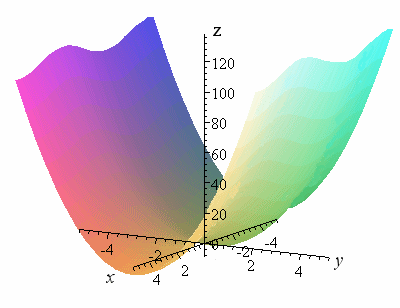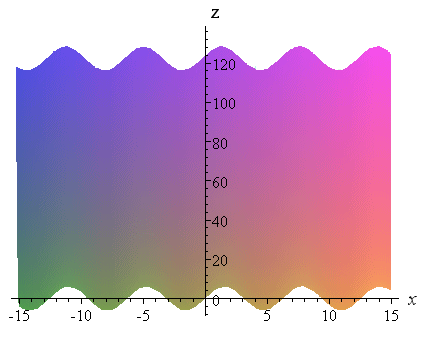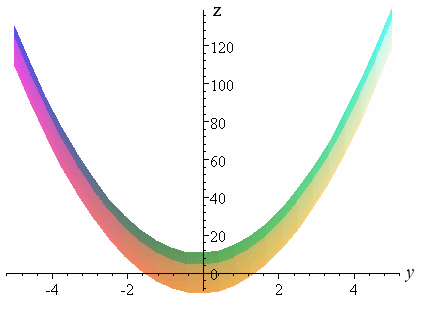Search IntMath
Close

450+ Math Lessons written by Math Professors and Teachers

5 Million+ Students Helped Each Year

1200+ Articles Written by Math Educators and Enthusiasts

Simplifying and Teaching Math for Over 23 Years

# 10. Partial Derivatives

by M. Bourne

So far in this chapter we have dealt with functions of single variables only. However, many functions in mathematics involve 2 or more variables. In this section we see how to find derivatives of functions of more than 1 variable.

This section is related to, but is not the same as Implicit Differentiation that we met earlier.

### Example 1 - Function of 2 variables

Here is a function of 2 variables, x and y:

F(x,y) = y + 6 sin x + 5y2

To plot such a function we need to use a 3-dimensional co-ordinate system.## Partial Differentiation with respect to x

"Partial derivative with respect to x" means "regard all other letters as constants, and just differentiate the x parts".

In our example (and likewise for every 2-variable function), this means that (in effect) we should turn around our graph and look at it from the far end of the y-axis. We are looking at the x-z plane only.We see a sine curve along the x-axis and this comes from the "6 sin x" part of our function F(x,y) = y + 6 sin x + 5y2. The y parts are regarded as constants (in fact, 0 in this case).

Now for the partial derivative of

F(x,y) = y + 6 sin x + 5y2

with respect to x:

(del F)/(del x)=6 cos x

The derivative of the 6 sin x part is 6 cos x. The derivative of the y-parts is zero since they are regarded as constants.

Notice that we use the curly symbol to denote "partial differentiation", rather than "d" which we use for normal differentiation.

NOTE: You can explore this example using this 3D interactive applet in the Vectors chapter. In the drop-down list of examples, this is the last one.

## Partial Differentiation with respect to y

The expression

Partial derivative with respect to y

means

"Regard all other letters as constants, just differentiate the y parts".

As we did above, we turn around our graph and look at it from the far end of the x-axis. So we see (and consider things from) the y-z plane only. (Notice the horizontal axis on the following graph is labeled y, not x.)

We see a parabola. This comes from the y2 and y terms in F(x,y) = y + 6 sin x + 5y2. The "6 sin x" part is now regarded as a constant. (It actually has the effect of moving the base of the parabola z=y+5y^2 down by 6 units.)Now for the partial derivative of

F(x,y) = y + 6 sin x + 5y2

with respect to y.

(delF)/(dely)=1+10y

The derivative of the y-parts with respect to y is 1 + 10y. The derivative of the 6 sin x part is zero since it is regarded as a constant when we are differentiating with respect to y.

NOTE: Once again, you can explore this particular example (rotate it, view it from different axes, etc) using the 3D interactive applet in the Vectors chapter. In the drop-down list of examples, select the last one.

## Second Order Partial Derivatives

We can find 4 different second-order partial derviatives. Let's see how this works with an example.

### Example 2

For the function we used above, F(x,y) = y + 6 sin x + 5y2, find each of the following:

(a) (del^2F)/(delydelx)

This could also be written as

del/(dely)[(delF)/(delx)]

This expression means:

"First, find the partial derivative with respect to x of the function F (this is in brackets), then find the partial derivative with respect to y of the result ".

In our example above, we found

(delF)/(delx)=6 cos x

To find (del^2F)/(delydelx), we need to find the partial derivative with respect to y of (delF)/(delx).

 (del^2F)/(delydelx)=del/(dely)[(delF)/(delx)]

=del/(dely)[6 cos x]

=0

Since cos x is a constant (when we are considering differentiation with respect to y), its derivative is just 0.

(b) (del^2F)/(delxdely)

This could also be written as

del/(delx)[(delF)/(dely)]

This expression means

Find the partial derivative with respect to x of the partial derivative with respect to y.

In our example above, F(x,y) = y + 6 sin x + 5y2, we found

(delF)/(dely)=1+10y

To find (del^2F)/(delxdely), we need to find the partial derivative with respect to x of (delF)/(dely).

(del^2F)/(delxdely)=del/(delx)[(delF)/(dely)]

=del/(delx)[1+10y]

=0

Since y is a constant (when we are considering differentiation with respect to x), its derivative is just 0.

(c) (del^2F)/(delx^2)

This could also be written as

del/(delx)[[delF)/(delx)]

This expression means

Find the partial derivative with respect to x of the partial derivative with respect to x.

In our example above, we found

(delF)/(delx)=6 cos x

To find (del^2F)/(delx^2), we need to find the derivative with respect to x of (delF)/(delx).

(del^2F)/(delx^2)=del/(delx)[[delF)/(delx)]

=del/(delx)[6 cos x]

=-6 sin x

(d) (del^2F)/(dely^2)

This could also be written as

del/(dely)[(delF)/(dely)]

This expression means:

Find the partial derivative with respect to y of the partial derivative with respect to y.

In our example above, F(x,y) = y + 6 sin x + 5y2, we found

(delF)/(dely)=1+10y

To find (del^2F)/(dely^2), we need to find the derivative with respect to y of (delF)/(dely).

 (del^2F)/(dely^2)=del/(dely)[(delF)/(dely)]

=del/(dely)[1+10y]

=10

## Problem SolverThis tool combines the power of mathematical computation engine that excels at solving mathematical formulas with the power of GPT large language models to parse and generate natural language. This creates math problem solver thats more accurate than ChatGPT, more flexible than a calculator, and faster answers than a human tutor. Learn More.